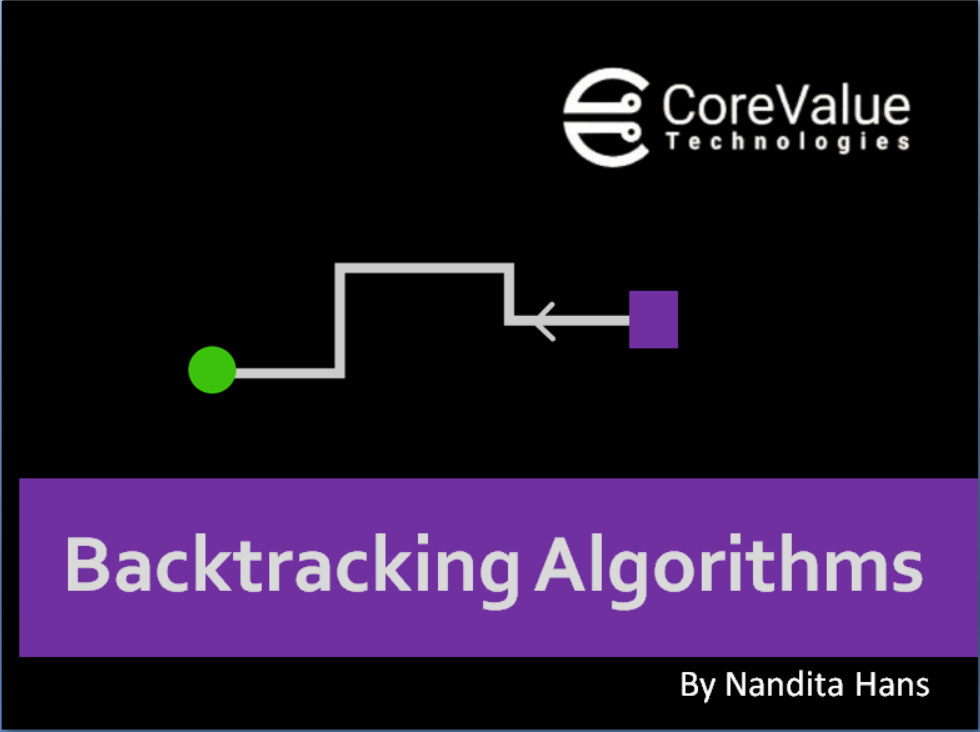Backtracking is a form of recursion. But it involves choosing only option out of any possibilities. We begin by choosing an option and backtrack from it, if we reach a state where we conclude that this specific option does not give the required solution. We repeat these steps by going across each available option until we get the desired solution.

This technique use brute force approach which says that for a given problem you should try all possible solutions and pick up desired solutions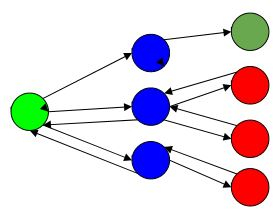Let's take a situation. Suppose you are standing in front of 3 doors, one of which is having correct path to your house, but you don't know which one. So you'll try all three. First open door 1, if that is not the one, close it, and open door 2, and again if that is not the one, close it and go into tunnel 3. So basically in backtracking we attempt solving a sub problem, and if we don't reach the desired solution, then undo whatever we did for solving that sub problem, and try solving another sub problem.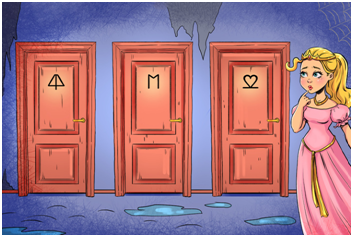# STANDARD BACKTRACKING PROBLEMS

## N QUEEN PROBLEM

The n queen’s puzzle is the problem of placing n chess queens on an n x n chessboard so that no two queens attack each other.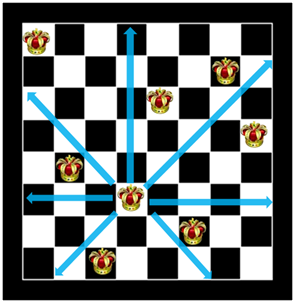Thus, a solution requires that no two queens share the same row, column, or diagonal.

Let’s now consider a 4 x 4 chessboard to understand this problem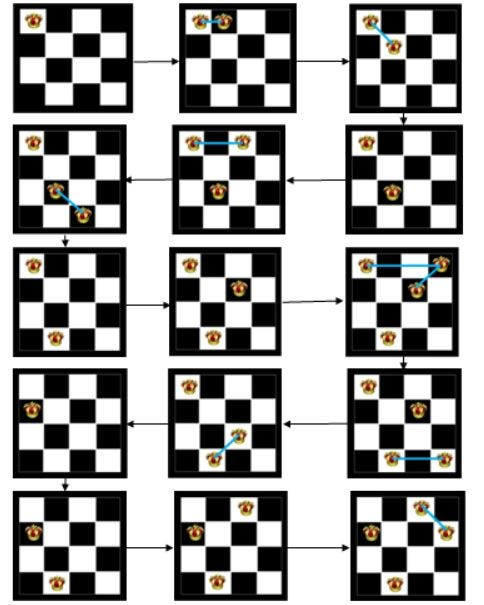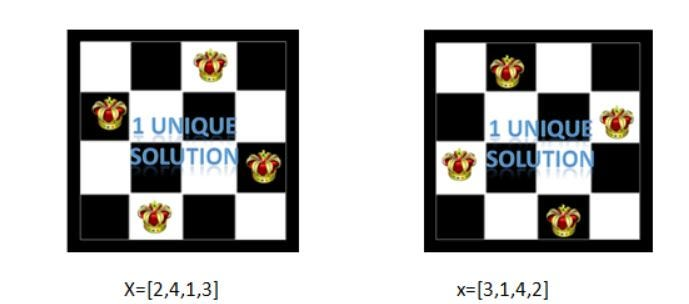These are the desired position of queens when we place them on 4x4 chess Board keeping the constraints in consideration that they are not in the same row,coloum and across the same diagonal.

We can similarly traverse it for a 8x8 Chessboard and will get these desired solutions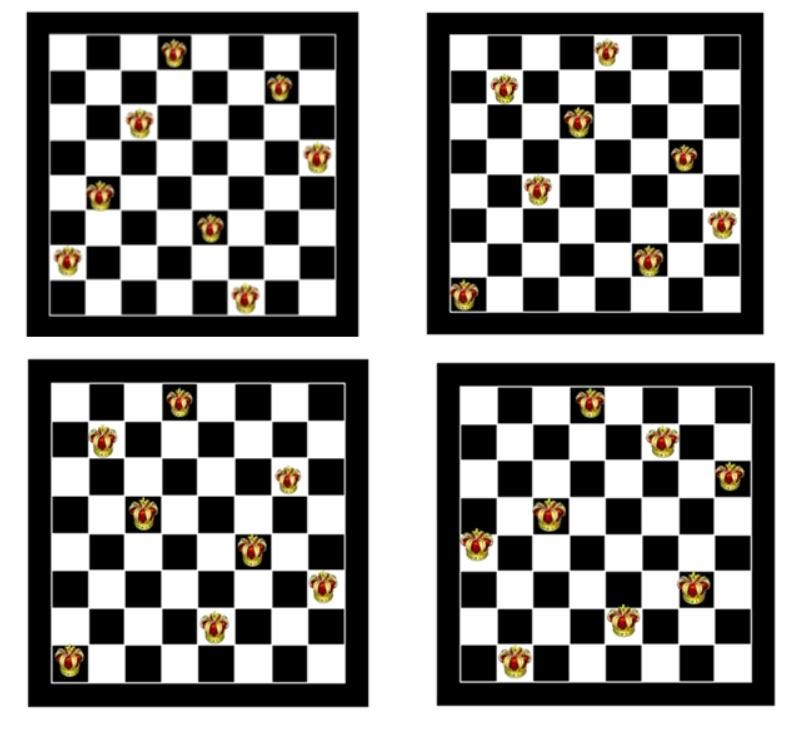### ALGORITHM

Now we have understand what N Queen Problem is let’s write a Algorithm for finding all the possible solution of placing the N Queen on an NxN  Chessboard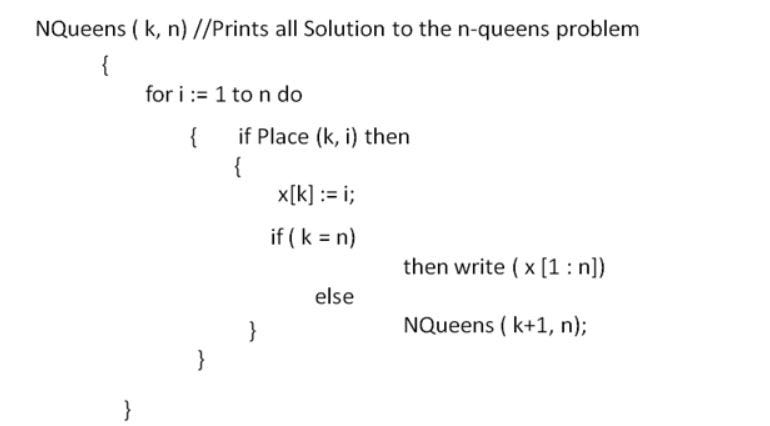The Place(k,i) function will check whether the Queen can be placed at that desired position of not such that it doesn’t lie along the same row,coloum or diagonal.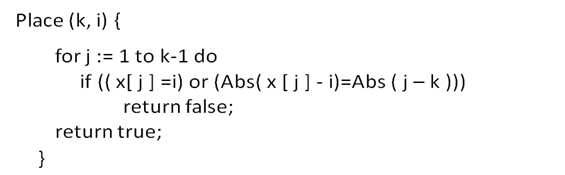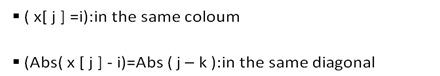Since we are traversing for each row we will now look whether Queens are not placed across the same coloum or diagonal.

### COMPLEXITY

Worst Case :“brute force” solution for the N-queens puzzle has an O(n^n) time complexity. This means it will look through every position on an NxN board, N times, for N queens.

brute force approach says that for a given problem you should try all possible solutions and pick up desired solutions

Best Case: The best case occurs if you find your solution before exploiting all possible arrangements. This depends on your implementation.

If we refactor and prevent it from checking queens occupying the same row as each other, it will still be brute force, and has a time complexity of O(n!).

## M COLORING DECISION PROBLEM

Given an undirected graph and a number m, determine if the graph can be colored with at most m colors such that no two adjacent vertices of the graph are colored with the same color. Here coloring of a graph means the assignment of colors to all vertices.

Foreg. We can take a graph with 4 vertices(n) and take out all possible way in which it can be colored with 3 colors(m).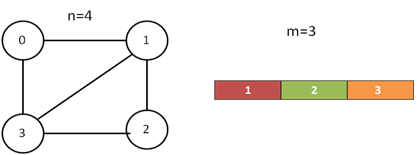Now,we have to color the graph in such a way such that no two adjacent vertices are of the same color. Now if we have given m=3 what are the possible way for colouring the graph.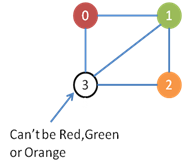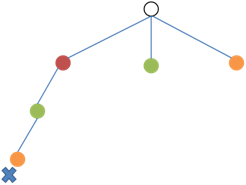We can construct a state space tree for looking into all the possible solutions and picking up the most desired solutions which satisfy all conditions of the bounding function.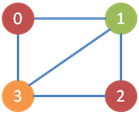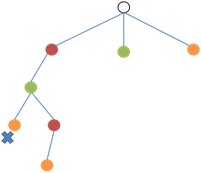We get one desired solution vector that is {Red,Green,Red,Orange} or{1,2,1,3}

Similarly we can traverse that State space tree and get the following desired solutions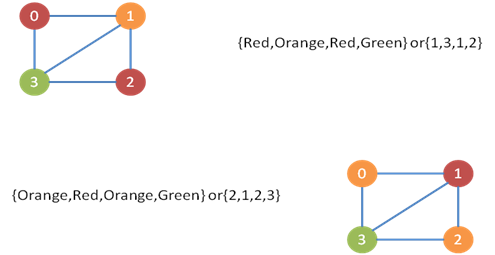### ALGORITHM

Construct an adjacency matrix for the graph to implement this algorithm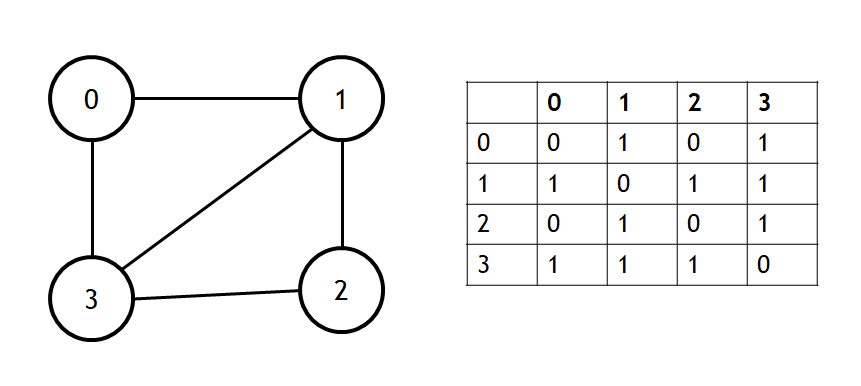Initially the solution vector is initialized with x=[0,0,0,0…….n]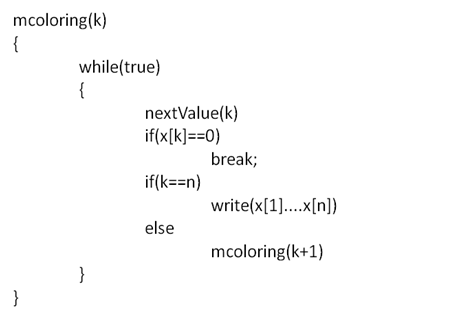When we call this function as mcoloring(1) and since it is a recursive call it will try for all possible vertex until the stack becomes empty.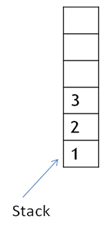The nextValue function will check whether it is possible to color the desired vertex with a next new color or next highest color. If it returns x[k]==0 it means no new color is possible for a given vertex.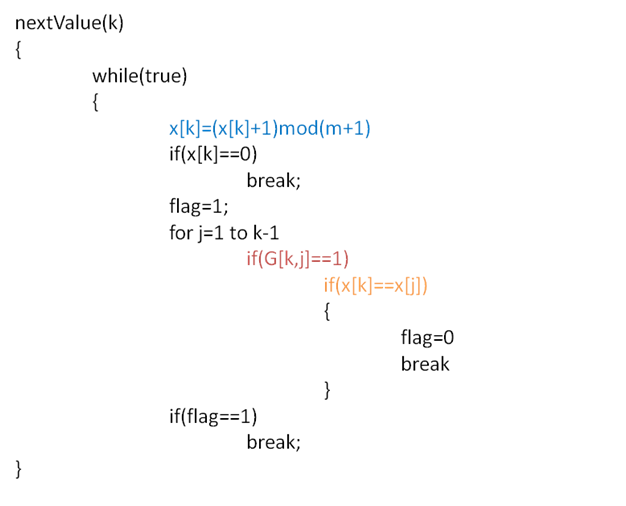x[k]=(x[k]+1)mod(m+1):

This will generate a new color each time or we can say next highest color

Intially x=(0,0,0,0) for graph with 4 vertices(n) and 3 colors(m)

So x[k]=(x[k]+1)mod(m+1)

x[k]=(0+1)mod(4)=1

x[k]=(1+1)mod(4)=2

x[k]=(2+1)mod(4)=3

x[k]=(3+1)mod(4)=0 means no new color is possible

if(G[k,j]==1):

It will check whether k and j are adjacent vertices.

if(x[k]==x[j]):

It will check if two adjacent vertex are of the same color.

### COMPLEXITY

Time Complexity: O(m^n).
There are total O(m^n) combination of colors. So time complexity is O(m^n). The upperbound time complexity remains the same but the average time taken will be less.

•  n is the number of vertices in graph
•  m which is the maximum number of colors that can be used.

Space Complexity: O(n).
To store the output array O(n) space is required.

Technologies